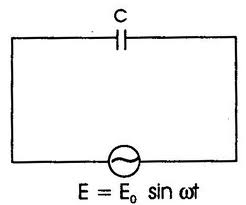## AC through Pure Capacitor Assignment Help

Assignment Help: >> Resistive - Inductive and Capacitive Circuits - AC through Pure Capacitor

AC through Pure Capacitor:

The current flowing through capacitor is given by

i = C dv /dt

= C dv / dt (Vm sin ωt )

= C Vm  ω cos ωt

Vm/(1/  ωC) sin( ωt +(π/2))

= Vm / XC   sin ( ωt +( π/2) )

Where  X c  = 1/ ωC  = Capacitive reactance in ohm.Figure : Pure Capacitive Circuit

If we put Im =     V m  / X C  , then

i = I m sin (ωt + (π/2) )

Here, we may see that current i leads the applied voltage by  π/2 .